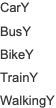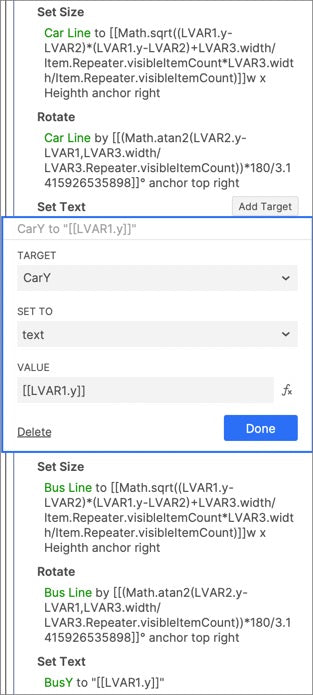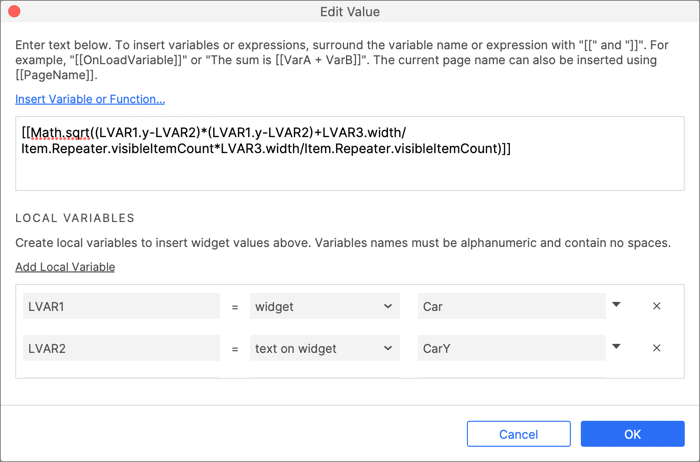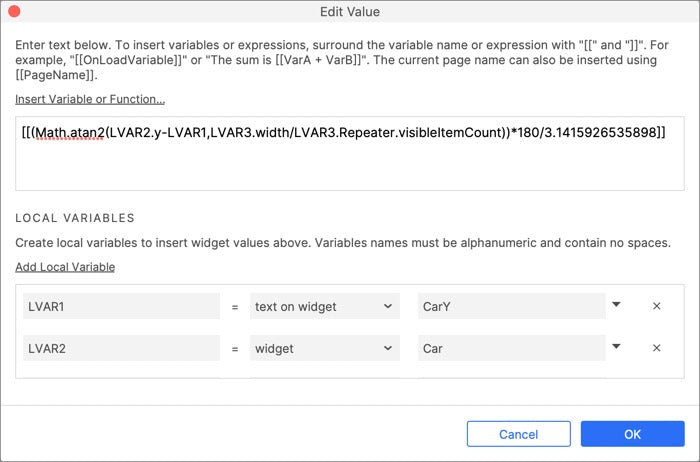# Axure Tutorial: Make Line Chart with Repeater

### Introduction

In Axure Tutorial: Making Scatter Chart with Repeater, We learned how to make scatter chart.

Today, let's learn how to make line chart, which is mainly used to view the trends of different categories. Line chart is made on the basis of scatter chart. Please learn to make scatter chart first.

· Simply fill in the repeater table to generate a line chart without connecting to the external network.

· The data hints can be shown when the mouse hover.

### Features to be Realized1. Automatically generate line chart after filling data in repeater table.

2. When the mouse enters dot, hint information will be shown.

### How to Realize these Features

1. Calculate the position, length and angle of the connecting line.

2. When the mouse enters the notice panel, the hints will be shown.

### Widgets SetupMake controls based on the scatter diagram, and add dotted lines next to each dot. Later, we will adjust the position, length and angle of the doted line to make line chart.Drag in 5 labels to save the y value of each dot.

### Interactions Setup

When the repeater item loaded, we need to save the y value of each dot into a label, which is used to calculate the difference between the Y values of line between two dots when the next repeater is loaded. Because the x value of each dot is the same when the item is loaded, the difference between the y value of the line between the two dots is equal to the width of the repeater divided by the number of visible items.

How to make a scatter chart will not be described here. Please refer to Axure Tutorial: Making Scatter Chart with Repeater. Here we mainly talk about how to set line.

#### Save Y value of dotAfter setting the size, position and angle of the finished line, you need to save its y value to the label.

#### Set Lines PositionMove the right side of all lines to the center of dots.

#### Set Lines Length

According to the formula of trigonometric function, the length of the line is equal to Sqrt((y2-y1) (y2-y1) + (x2-x1) (x2-x1)).Y2-y1 is equal to the y value of dot - the y value of the previous item saved in the label. X2-y1 equals the width of the repeater divided by the number of visible items.

#### Set the Rotation Angle of the LinesBased on the tangent value, the angle of rotation to the line can be calculated. The function of Axure is [[(Math.atan2 ((y2-y1), (x2-x1)) *180 / 3.1415926535898)]], and the rotating anchor point is in the middle of the right side.

####Select the line in repeater, when loaded, if Item.index equals 1, it needs to be set as hidden.

If you like the article, please share it with others with page link, thanks for your supporting! ❤

Well Joe @AxureBoutique, a technical writer and teacher, focuses on Axure prototype design and product design.